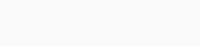# Polymath 6.10.260 Professional

### Polymath

POLYMATH is a proven computational system that has been specifically created for educational or professional use. The various POLYMATH programs allow the user to apply effective numerical analysis techniques during interactivate problem solving on personal computers. Results are presented graphically for easy understanding and for incorporation into papers and reports. Engineers, mathematicians, scientists, students, or anyone with a need to solve problems will appreciate the efficiency and speed of problem solution.

POLYMATH utilizes a color-coded full-screen editor.  All displays have been optimized for user efficiency.  Also provided with Polymath 6.1 is the UNIQUE capability to automatically export any POLYMATH problem to Excel with a single keypress. Thus problems can be solved completely in Polymath or exported to ExcelTM for solution. A POLYMATH ODE_Solver Add-In is included for solving ordinary differential equations in Excel. Automatic export to Excel includes all intrinsic functions and logical variables. Ordered equations can also be provided to assist with optional MatlabTM solutions of POLYMATH problems.

The Professional Version problem-solving capabilities include:

• Linear Equations – up to 264 simultaneous equations
• Nonlinear Equations – up to 300 simultaneous nonlinear and 300 additional explicit algebraic equations
• Differential Equations – up to 300 simultaneous ordinary differential and 300 additional explicit algebraic equations
• Data analysis and Regression – up to 200 variables with up to 1000 data points for each, with capabilities for linear, multiple linear, and nonlinear regressions with extensive statistics plus polynomial and spline fitting with interpolation and graphing capabilities